• Call Now

1800-102-2727•

# Faraday's law of electromagnetic induction-definition,types, practice problems, FAQs

Sarvesh goes to the ATM to draw some money. He inserts his debit card into the machine. He noticed that as soon as the chip portion of the card went in, the machine showed relevant information on the screen needed to draw cash. He also owns a laptop on which he frequently records games; the information he needs is stored in the hard disk in the form of binary digits 0 and 1. The science behind these two is a phenomenon called “electromagnetic induction”. A scientist named Faraday, around 1831, conducted an experiment with a magnet and a conducting coil. He noticed that when the magnet was moved keeping the coil stationary, a current was produced in the coil. Current was also produced when the coil was rotated keeping the magnet stationary. From this, he was able to conclude that electricity and magnetism share a close relation. Now, how does this relate to the debit card swiped at an ATM? The machine’s wires cause a change in magnetic field which produces a current that relays Sarvesh’s card’s details to the machine. Hard disks contain a spinning disk with a magnetic layer that store information in binary language (0 and 1). Many other equipment like magnetic brakes, induction cookers, generators work on the same principle of electromagnetic induction. So let’s explore Faraday’s law in detail.

• Definition of magnetic flux
• Different cases of magnetic flux
• Faraday’s first law of electromagnetic induction
• Faraday’s second law of electromagnetic induction
• Induced charge
• Practice problems
• FAQs

## Definition of Magnetic Flux

Before we understand Faraday’s law, we must know the concept of magnetic flux. The number of magnetic lines of force crossing a given area held perpendicular to the surface (area vector) is called the magnetic flux. Let $\stackrel{\to }{B}$ indicate the magnetic field and A indicate the area vector of a coil; The magnetic flux$\left(\varphi \right)$ linked with the coil can be written as dot product of $\stackrel{\to }{B}$ and A. i.e =where is the angle between $\stackrel{\to }{B}$ and $\stackrel{\to }{A}$.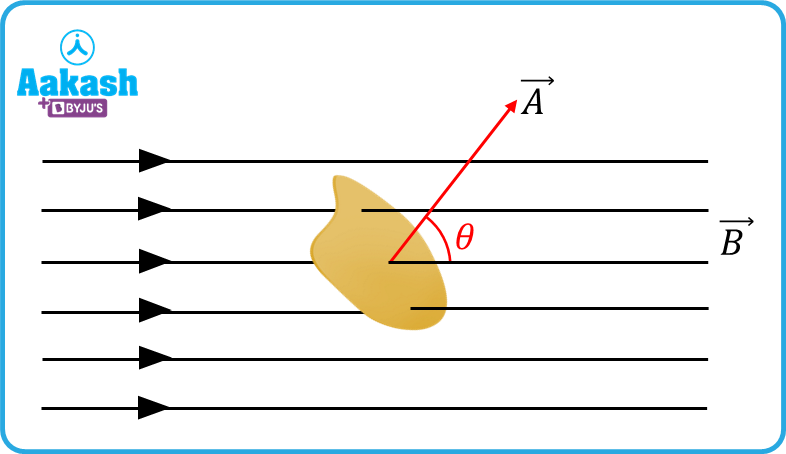Different cases of magnetic flux

(i) When the coil is held perpendicular to the direction of $\stackrel{\to }{B}$, angle between $\stackrel{\to }{B}$ and $\stackrel{\to }{A}$ is $\theta ={0}^{0}.$ Then flux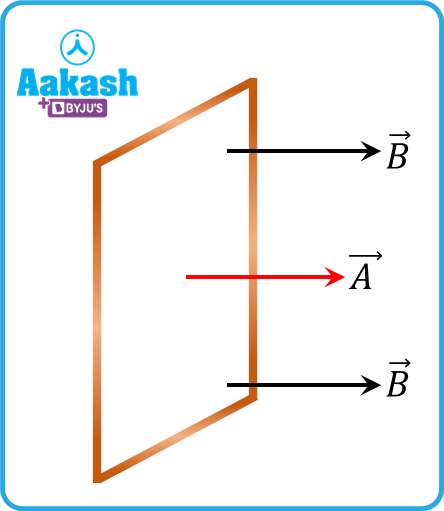(ii)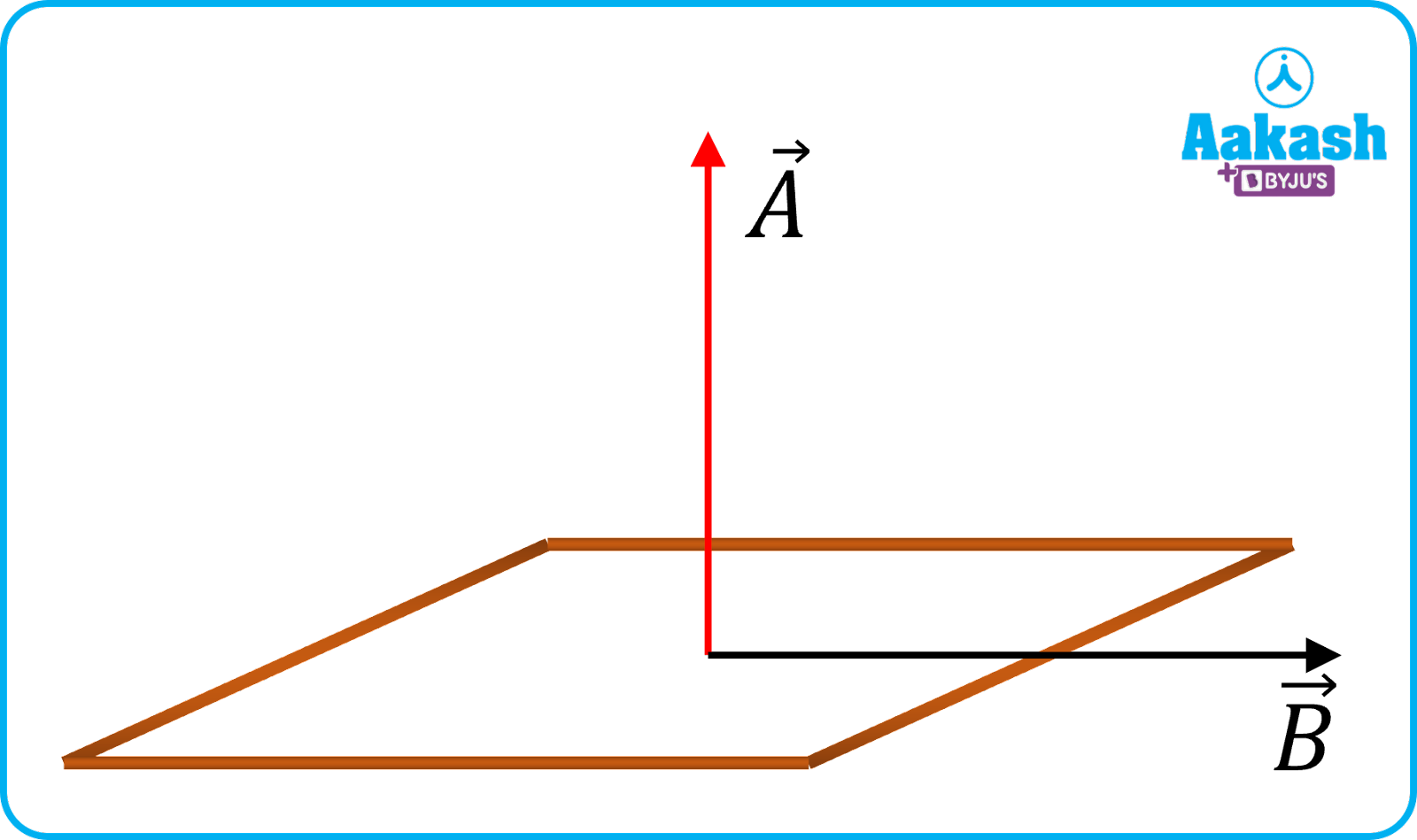(iii) When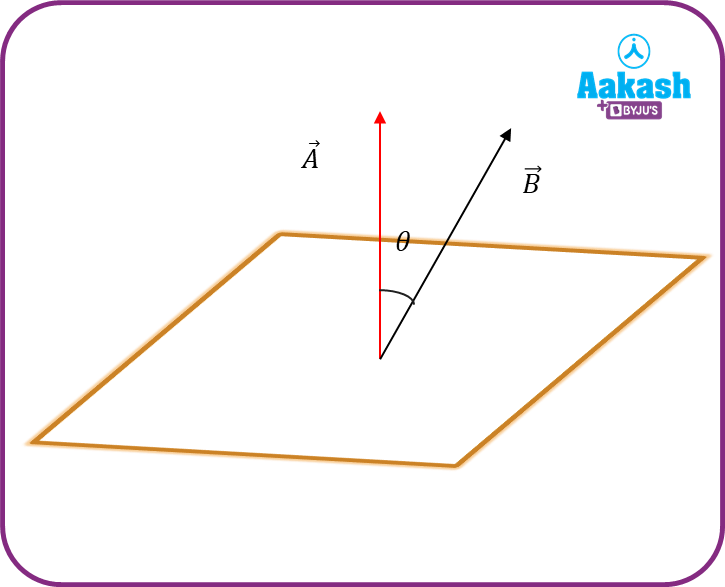(iv) When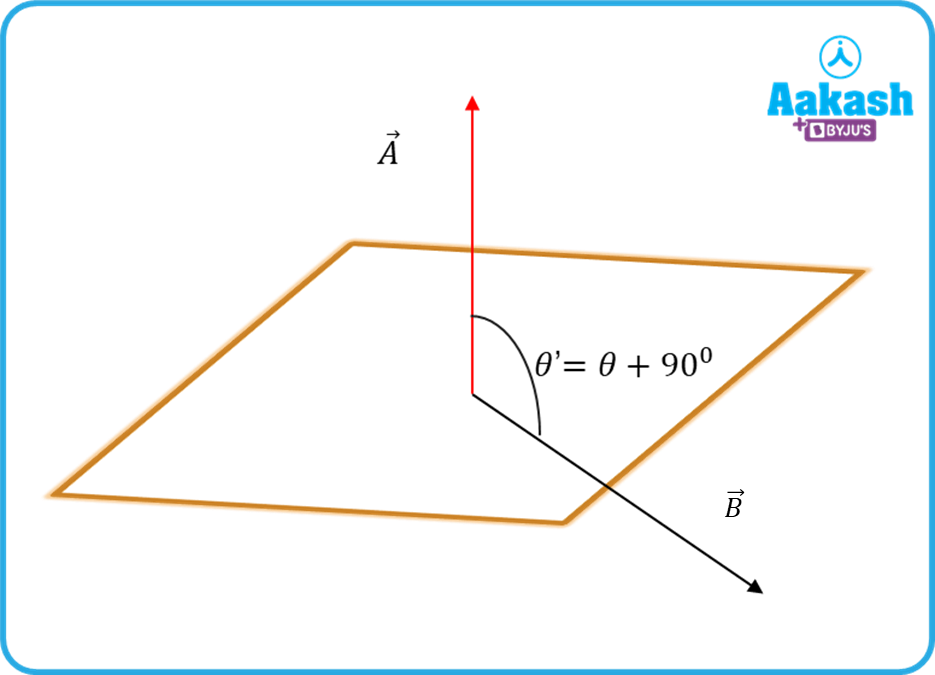(v) When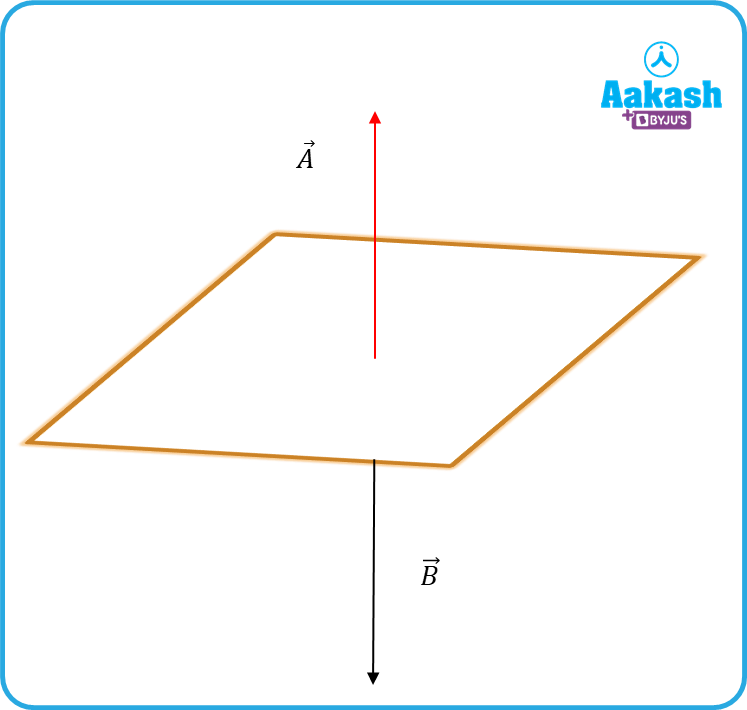Faraday’s first law of electromagnetic induction

Whenever the magnetic flux linked with a coil changes, an emf is induced in the coil.

While experimenting with a bar magnet and a coil connected to a galvanometer; Faraday noticed that whenever the magnet was moved, the galvanometer showed a deflection. He called this connection between current and magnetism as “ electromagnetism”.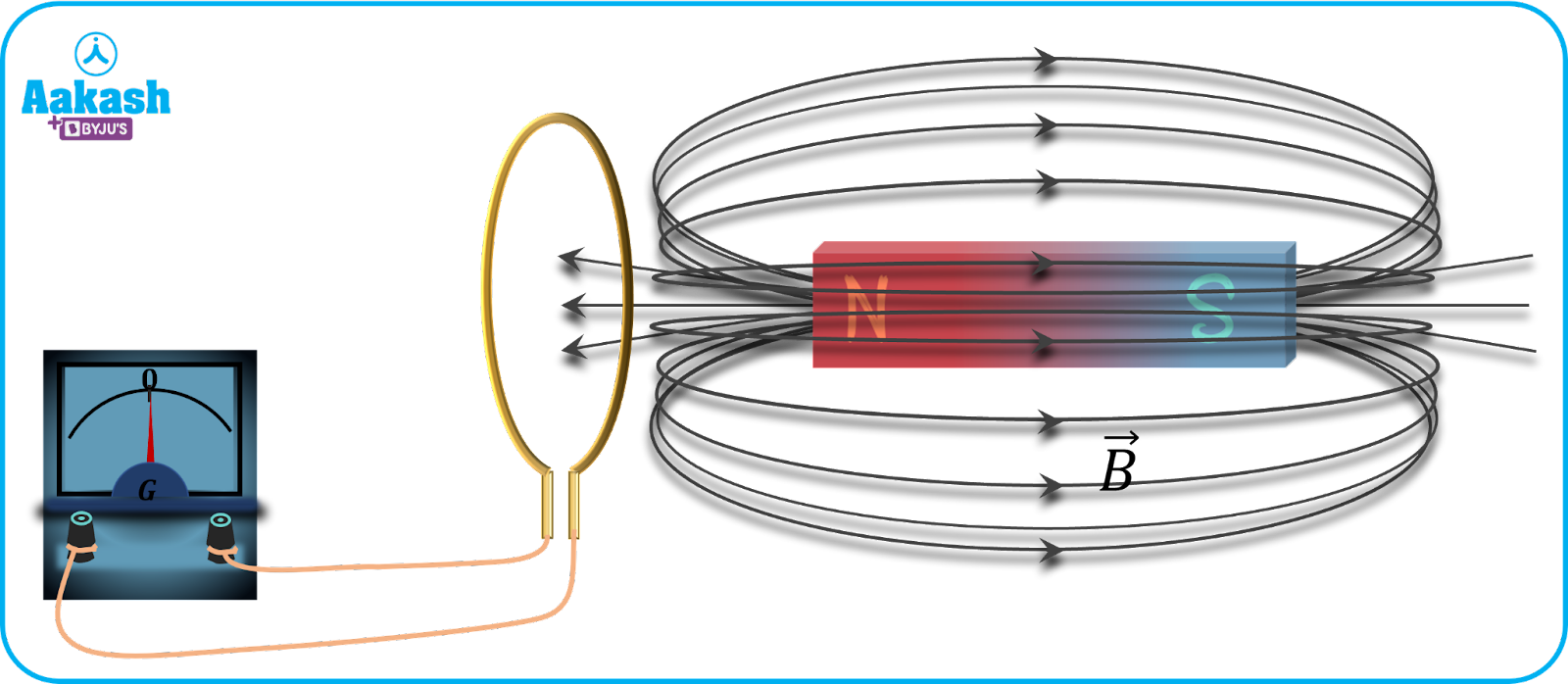## Faraday’s second law of electromagnetic induction

The magnitude of the induced emf is equal to the rate of change of magnetic flux associated with the coil.

$\epsilon =-\frac{d\varphi }{dt}---\left(i\right)$

The negative sign indicates that the coil opposes the magnetic field change.

$\left|\epsilon \right|=\left|\frac{d\varphi }{dt}\right|$

Let $\stackrel{\to }{A}$ indicate the cross sectional area of the conductor coil and B be the magnetic field. If N indicates the number of turns, then the flux linked with the coil,

$\varphi =NBAcos\theta --\left(ii\right)$

Substituting equation (ii) in equation (i), we get

$\epsilon =-\frac{d\left(NBAcos\theta \right)}{dt}--\left(iii\right)$

Now if the coil is rotated keeping the magnetic stationary, then =⍵t

⍵ indicates the angular velocity of the coil.

So, Equation (iii) becomes

$\epsilon =-d\left(NBAcos⍵t\right)dt=NB⍵Asin⍵t$

The induced current i produced in the coil, $i=\frac{\epsilon }{R}$

Where, R indicates the resistance of the coil.

indicates the peak value of current.

In the above case, B indicates a constant magnetic field where the coil rotates.

When the magnetic field varies with time, equation (iii) becomes

$\epsilon =-\frac{d\left(NBAcos\theta \right)}{dt}=-NAcos\theta \frac{dB}{dt}$

$\frac{dB}{dt}$indicates change in magnetic field with respect to time.

## Induced charge

As a result of current induced in the circuit, charge also starts flowing in it. Let dq be the charge flowing in time dt. Then,

dq=idt,

$i=\frac{e}{R},e=-\frac{d\varphi }{dt}⇒i=-\frac{1}{R}\frac{d\varphi }{dt}$

Therefore, the charge flown becomes, $dq=-\frac{1}{R}\frac{d\varphi }{dt}×dt=-\frac{1}{R}×d\varphi$

$dq=-\frac{d\varphi }{R}$

If a coil having N turns, cross sectional area A is rotated from an angle i to an angle f in a magnetic field B, the charge flown becomes

$\mathrm{\Delta q}=\frac{NBA\left(cos{\theta }_{i}-cos{\theta }_{f}\right)}{R}$

Practice problems

Q. A coil having cross-sectional area 1 m2, resistance 10 and number of turns 100 is placed with its plane perpendicular to a magnetic field which varies according to the relation, B=2t2, where t is the instantaneous time. Calculate

(i) the induced emf

(ii) the induced current at t=1 s

A. Given,

(i) induced emf,

The induced emf at

(ii) induced current,$\frac{\epsilon }{R}=\frac{4t}{10}$

Maximum induced emf at t=1 s

Q. The magnetic flux across a loop of resistance 10 varies as a function of time given by =10t2-2t+1 Wb. How much current is induced in the loop after 0.3 s ?

A. a

We know,

$=\left|\frac{d\varphi }{dt}\right|=\frac{d}{dt}\left(10{t}^{2}-2t+1\right)=\left(20t-2\right)$

Q. A coil having an area of A0 is placed in a magnetic field which changes from B0 to 5B0 in time interval t. The emf induced in the coil will be,

A. b

The magnitude of induced emf $\epsilon =\frac{d\varphi }{dt}=\frac{d\left(BA\right)}{dt}={A}_{0}\left(\frac{{5B}_{0}-{B}_{0}}{t}\right)=\frac{4{A}_{0}{B}_{0}}{t}$

Q. How much charge will flow through the ring having a diameter of 10 cm and a resistance of 0.01 ., if it is initially held perpendicular to the magnetic field of intensity of 1 T and then rotated to bring it parallel to the direction of the field ?

A.b

Let dq be the charge flown. Then $dq=-\frac{d\varphi }{R}$

Given,

Flux change

## FAQs

Q. In Faraday's experiment, if both the coil and the magnet are moved at the same speed, no emf is induced. Why?
A.
When both the coil and the magnet are moved with the same speed, the relative speed of the coil with respect to the magnet is zero. In that case, no flux change linked with the coil happens which means no emf will be induced.

Q. How’s Faraday’s law different from Lenz’s law?.
A.
Michael Faraday experimented with coil and magnet and proved that a varying magnetic flux produces an emf – thereby an induced current. He stated that greater the change in magnetic flux, more the induced current. Lenz’s law talks about the direction of induced current and explains it from the point of conservation of energy.

Q. Give one application of electromagnetic induction?
A.
Electromagnetic induction is used in ATM cards where a small magnetic field is produced in the chip of the card when it is inserted into the machine. This interacts with a small current pulse in the ATM, signaling necessary information and to dispense cash.

Q. Is Faraday’s law a consequence of conservation of energy?
A.
No. Faraday’s law only talks about induced current when there is a magnetic flux change. It does not give any idea regarding where this electrical current comes from. Faraday's law just links magnetism with electricity.Talk to our expert
Resend OTP Timer =
By submitting up, I agree to receive all the Whatsapp communication on my registered number and Aakash terms and conditions and privacy policy